# CBSE Question Paper 2012 class 12 Chemistry## myCBSEguide App

CBSE, NCERT, JEE Main, NEET-UG, NDA, Exam Papers, Question Bank, NCERT Solutions, Exemplars, Revision Notes, Free Videos, MCQ Tests & more.

CBSE Question Paper 2012 class 12 Chemistry conducted by Central Board of Secondary Education, New Delhi in the month of March 2012. CBSE previous year question papers with the solution are available in the myCBSEguide mobile app and website. The Best CBSE App for students and teachers is myCBSEguide which provides complete study material and practice papers to CBSE schools in India and abroad.

CBSE Question Paper 2012 class 12 Chemistry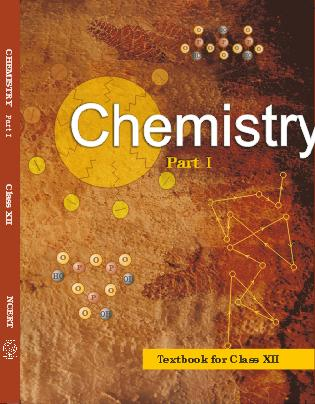## Class 12 Chemistry list of chapters

1. The Solid State
2. Solutions
3. Electrochemistry
4. Chemical Kinetics
5. Surface Chemistry
6. General Principles and Processes of Isolation of Elements
7. The p-Block Elements
8. The d and f Block Elements
9. Coordination Compounds
10. Haloalkanes and Haloarenes
11. Alcohols, Phenols and Ethers
12. Aldehydes, Ketones and Carboxylic Acids
13. Amines
14. Biomolecules
15. Polymers
16. Chemistry in Everyday life

## CBSE Question Paper 2012 class 12 Chemistry

### General Instructions

1. All questions are compulsory.
2. Marks for each question are indicated against it.
3. Question numbers 1 to 8 are very short-answer questions, carrying 1 mark each. Answer these in one word or about one sentence each.
4. Question numbers 9 to 18 are short-answer questions, carrying 2 marks each. Answer these in about 30 words each.
5. Question numbers 19 to 27 are short-answer questions of 3 marks each. Answer these in about 40 words each.
6. Question numbers 28 to 30 are long-answer questions of 5 marks each. Answer these in about 70 words each.
7. Use Log Tables, if necessary Use of calculators is not permitted.

### CHEMISTRY 2012 (Delhi)

1. Write a point of distinction between a metallic solid and an ionic solid other than metallic lustre. 

2. Which one of PCl4+ and PCl4 is not likely to exist and why? 

3. What is the role of graphite in the electrometallurgy of aluminium? 

4. Arrange the following compounds in an increasing order of their reactivity in nucleophilic addition reactions: ethanal, propanal, propanone, butanone. 

5. Draw the structural formula of 2-methylpropan-2-ol molecule. 

6. Give the IUPAC name of the following compound.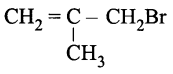7. Define the term, “homopolymerisation giving an example. 

8. Arrange the following in the decreasing order of their basic strength in aqueous solutions: CH3NH2, (CH3)2NH,(CH3)3N and NH3

9. A 1.00 molal aqueous solution of trichloroacetic acid (CCl3COOH) is heated to its boiling point. The solution has the boiling point of 100.18oC. Determine the vant Hoff factor for trichloroacetic acid. (Kb for water = 0.512K kg mol-1

### OR

Define the following terms:
(i) Mole fraction
(ii) Isotonic solutions
(iii) Van ‘t Hoff factor
(iv) Ideal solution

10. What do you understand by the “order of a reaction? Identify the reaction order from each of the following units of reaction rate constant: 
i) L-1 mols-1
ii) L mol-1s-1

11. Describe a conspicuous change observed when 
(i) a solution of NaCl is added to a sol of hydrated ferric oxide.
(ii) a beam of light is passed through a solution of NaCl and then through a sol.

12. What is meant by coagulation of a colloidal solution? Describe briefly any three methods by which coagulation of lyophobic sols can be carried out. 

13. Describe the following: 
(i) The role of cryolite in electro metallurgy of aluminium.
(ii) The role of carbon monoxide in the refining of crude nickel.

14. What is meant by (i) peptide linkage (ii) biocatalysts? 

15. Explain the following giving an appropriate reason in each case. 
(i) O2 and F2 both stabilize higher oxidation states of metals but O2 exceeds F2 in doing so.
(ii) Structures of Xenon fluorides cannot be explained by Valence Bond approach.

16. Complete the following chemical equations: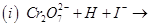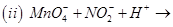17. Draw the structure of the monomer for each of the following polymers: 
(i) Nylon 6
(ii) Polypropene

18. Write the main structural difference between DNA and RNA. Of the two bases, thymine and uracil, which one is present in DNA? 

19. Tungsten crystallizes in body centred cubic unit cell. If the edge of the unit cell is 316.5 pm, what is the radius of tungsten atom? 

### OR

Iron has a body centred cubic unit cell with a cell dimension of 286.65pm. The density of iron is 7.874g cm-3.Use this information to calculate Avogadro’s number. (At mass of Fe=55.845u)

20. A solution of glycerol (C3H8O3) in water was prepared by dissolving some glycerol in 500g of water. This solution has a boiling point of 100.42oC while pure water boils at 100 °C. What mass of glycerol was dissolved to make the solution?(Kb for water = 0.512K kg mol-1

21. For the reaction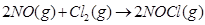the following data were collected. All the measurements were taken at 263 K:

 Experiment No. Initial [NO](M) Initial [Cl2](M) Initial rate of disappearance of Cl2(M/min) 1 0.15 0.15 0.60 2 0.15 0.30 1.20 3 0.30 0.15 2.40 4 0.25 0.25 ?

(a) Write the expression for rate law.
(b) Calculate the value of rate constant and specify its units.
(c) What is the initial rate of disappearance of Cl2 in exp. 4?

22. State a reason for each of the following situations: 
(i) Co2+ is easily oxidized to Co3+ in presence of a strong ligand.
(ii) CO is a stronger complexing reagent than NH3.
(iii) The molecular shape of Ni(CO)4 is not the same as that of [Ni(CN)4]2-.

23. How would you account for the following? 
(i) With the same d-orbital configuration (d4) Cr2+ is a reducing agent while Mn3+ is an oxidizing agent.
(ii) The actinoids exhibit a larger number of oxidation states than the corresponding members in the lanthanoid series.
(iii) Most of the transition metal ions exhibit characteristic in colours in aqueous solutions.

24. Write chemical equations for the following conversions: 
(i) Nitrobenzene to benzoic acid.
(ii) Benzyl chloride to 2-phenylethanamine.
(iii) Aniline to benzyl alcohol.

25. What are the following substances? Give one example of each one of them. 
(i) Tranquilizers
(ii) Food preservatives
(iii) Synthetic detergents

26. Draw the structure and name the product formed if the following alcohols are oxidized. Assume that an excess of oxidizing agent is used. 
(i) CH3CH2CH2CH2OH
(ii) 2-butenol
(iii) 2-methyl-1-propanol

27. Although chlorine is an electron withdrawing group, yet it is ortho-, para-directing in electrophilic aromatic substitution reactions. Explain why it is so? 

28. (a) Complete the following chemical reaction equations: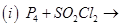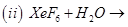(b) Predict the shape and the asked angle (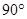or more or less) in each of the following cases: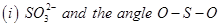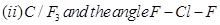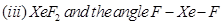### OR

(a) Complete the following chemical equations: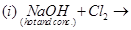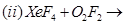(b) Draw the structures of the following molecules: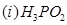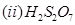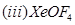29. (a) What type of a battery is the lead storage battery? Write the anode and the cathode reactions and the overall reaction occurring in a lead storage battery when current is drawn from it.
(b) In the button cell, widely used in watches, the following reaction takes place
Zn(s) + Ag2O(s)+ H2O(l)→→Zn2+(aq)+2Ag(s)+2OH(aq)
Determine Eo and △△G0 from the reaction.(given: EoAg+/Ag = +0.80V, E2+Zn2+/Zn = -0.76V) 

### OR

(a) Define molar conductivity of a solution and explain how molar conductivity changes with change in concentration of solution for a weak and a strong electrolyte.
(b) The resistance of a conductivity cell containing 0.001M KCl solution at 298K is 1500 ππ  What is the cell constant if the conductivity of 0.001M KCl solution at 298 K is 0.146×10-3 S cm-1?

30. (a)Give a plausible explanation for each one of the following: 
(i) There are two -NH2 groups in semicarbazide. However, only one such group is involved in the formation of semicarbazones.
(ii) Cyclohexanone forms cyanohydrin in good yield but 2, 4, 6-trimethylcyclohexanone does not.
(b) An organic compound with molecular formula C9H10O forms 2, 4, – DNP derivative, reduces Tollens reagent and undergoes Cannizzaro’s reaction. On vigorous oxidation it gives 1, 2-benzene-di-carboxylic acid. Identify the compound.

### OR

(a) Give chemical tests to distinguish between
(i) Phenol and Benzoic acid
(ii) Benzophenone and Acetophenone
(b) Write the structures of the main products of following reactions: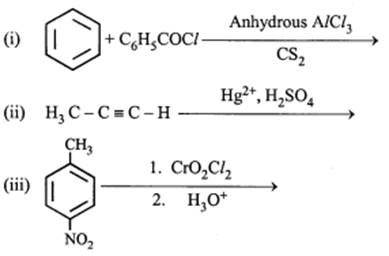These are questions only. To view and download complete question paper with solution install myCBSEguide App from google play store or login to our student dashboard.

## Chemistry Question Paper 2012

Download class 12 Chemistry question paper with solution from best CBSE App the myCBSEguide. CBSE class 12 Chemistry question paper 2012 in PDF format with solution will help you to understand the latest question paper pattern and marking scheme of the CBSE board examination. You will get to know the difficulty level of the question paper. CBSE question papers 2012 for class 12 Chemistry have 30 questions with solution.

## Previous Year Question Paper for class 12 in PDF

CBSE question papers 2018, 2017, 2016, 2015, 2014, 2013, 2012, 2011, 2010, 209, 2008, 2007, 2006, 2005 and so on for all the subjects are available under this download link. Practicing real question paper certainly helps students to get confidence and improve performance in weak areas.

To download CBSE Question Paper 2012 class 12 Accountancy, Chemistry, Physics, History, Political Science, Economics, Geography, Computer Science, Home Science, Accountancy, Business Studies and Home Science; do check myCBSEguide app or website. myCBSEguide provides sample papers with solution, test papers for chapter-wise practice, NCERT solutions, NCERT Exemplar solutions, quick revision notes for ready reference, CBSE guess papers and CBSE important question papers. Sample Paper all are made available through the best app for CBSE students and myCBSEguide website.